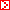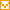ENGLISHHOMEプロジェクト概要組織図概念図スタッフ研究成果ディスカッションペーパーデータベースお知らせ公募情報研究会日程過去の研究会と報告資料レクチャーシリーズ過去のレクチャーと報告資料ニュースレターリンク一橋大学一橋大学附属図書館一橋大学経済研究所社会科学統計情報研究センターアジア長期経済統計プロジェクトGlobal Economic History Network政府統計ミクロデータの試行的提供ICPSR データアーカイブAMU and AMU Deviation indicatorsAsymptotic Properties of the Efficient Estimators for Cointegrating Regression Models with Serially Dependent Errors

 Eiji Kurozumi and Kazuhiko Hayakawa

 December, 2006

 Abstract
 In this paper, we analytically investigate three efficient estimators for cointegrating regression models: Phillips and Hansen's (1990) fully modified OLS estimator, Park's (1992) canonical cointegrating regression estimator, and Saikkonen's (1991) dynamic OLS estimator. First, by the Monte Carlo simulations, we demonstrate that these efficient methods do not work well when the regression errors are strongly serially correlated. In order to explain this result, we assume that the regression errors are generated from a nearly integrated autoregressive (AR) process with the AR coefficient approaching 1 at a rate of 1/T , where T is the sample size. We derive the limiting distributions of the three efficient estimators as well as the OLS estimator and show that they have the same limiting distribution under this assumption. This implies that the three efficient methods no longer work well when the regression errors are strongly serially correlated. Further, we consider the case where the AR coefficient in the regression errors approaches 1 at a rate slower than 1/T . In this case, the limiting distributions of the efficient estimators depend on the approaching rate. If the rate is slow enough, the efficiency is established for the three estimators; however, if the approaching rate is relatively fast, they have the same limiting distribution as the OLS estimator. This result explains why the effect of the efficient methods diminishes as the serial correlation in the regression errors gets stronger.Copyright (C) 2003-2007 by Institute of Economic Research.All rights reserved.